## NCERT Solutions for Class 9 Maths Chapter 13 Surface Areas and Volumes Ex 13.6

NCERT Solutions for Class 9 Maths Chapter 13 Surface Areas and Volumes Ex 13.6 are part of NCERT Solutions for Class 9 Maths. Here we have given NCERT Solutions for Class 9 Maths Chapter 13 Surface Areas and Volumes Ex 13.6.

 Board CBSE Textbook NCERT Class Class 9 Subject Maths Chapter Chapter 13 Chapter Name Surface Areas and Volumes Exercise Ex 13.6 Number of Questions Solved 8 Category NCERT Solutions

## NCERT Solutions for Class 9 Maths Chapter 13 Surface Areas and Volumes Ex 13.6

Question 1.
The circumference of the base of a cylindrical vessel is 132 cm and its height is 25 cm. How many litres of water can it hold? (1000cm3 =1 L.)
Solution:
We have, circumference of the base = 132 cm
∴ 2πr = 132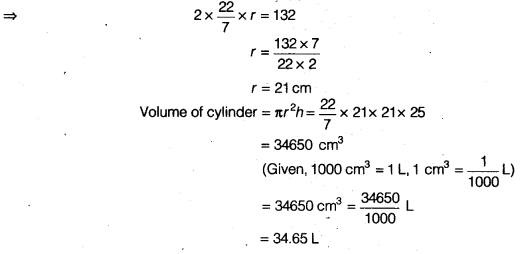Question 2.
The inner diameter of a cylindrical wooden pipe is 24 cm and its outer diameter is 28 cm. The length of the pipe is 35 cm. Find the mass of the pipe, if 1 cm3 of wood has a mass of 0.6 g.
Solution:
Given, inner diameter = 24 cm
Outer diameter = 28 cm
h = 35cmQuestion 3.
A soft drink is available in two packs
(i) a tin can with a rectangular base of length 5 cm and width 4 cm, having a height of 15 cm.
(ii) a plastic cylinder with circular base of diameter 7 cm and height 10 cm. Which container has greater capacity and by how much?
Solution:
(i) We have, h = 15cm
l = 5cm, b = 4cm, h = 15 cm
Volume of cuboidical body =l x b x h= 5 x 4 x 15 = 300cm3 …(i)
(ii) We have, Diameter = 7cm
Radius, r = $$\frac { 7 }{ 2 }$$ cm
Height, h = 10cm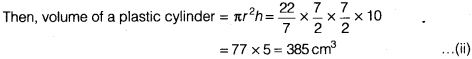∴ From Eqs. (i) and (ii), we see that volume of a plastic cylinder has greater capacity and its capacity is 385 – 300 = 85 cm3 is more than the tin can.

Question 4.
If the lateral surface of a cylinder is 94.2 cm2 and its height is 5 cm, then find
(ii) its volume. (Use π = 3.14)
Solution:
We have, lateral surface of a cylinder = 94.2 cm2 and h = 5 cm
∴ 2πrh = 94.2
⇒ 2 x 3.14 x r x 5 = 94.2
⇒ r = $$\frac { 94.2 }{ 31.4 }$$
⇒ r = 3 cm
(i) Hence, radius of base, r = 3cm
(ii) Volume of a cylinder = πr2h= 3.14(3)2x 5
= 3.14 x 9 x 5
= 141.3 cm3

Question 5.
It costs ₹2200 to paint the inner curved surface of a cylindrical vessel 10 m deep. If the cost of painting is at the rate of ₹20 per m2, find
(i) inner curved surface area of the vessel,
(iii) capacity of the vessel.
Solution:
We have, cost to paint the inner curved surface = ₹2200
Cost to paint per m2 = ₹20(i) Inner curved surface area of the vessel = 110m2
(ii) Hence, radius of the base is 1.75 m.
(iii) Capacity of the vessel = Volume of the vesselQuestion 6.
The capacity of a closed cylindrical vessel of height 1 m is 15.4 litres. How many square metres of metal sheet would be needed to make it?
Solution:
Capacity of a closed cylindrical vessel = 15.4 L
= 15.4 x 1000cm3 (∵ 1L = 1000 cm2)
∴ πr2h = 15400 cm3
πr2 x 100 = 15400
r2 = 154 x $$\frac { 7 }{ 22 }$$ (∵ h= 1m= 100cm)
⇒ r2 = 7 x 7
⇒ r = 7 cm
Total surface area of closed cylindrical vessel = 2πr(r + h)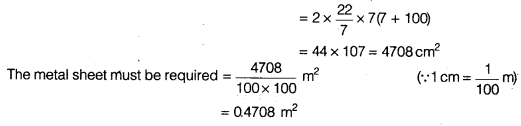Question 7.
A lead pencil consists of a cylinder of wood with a solid cylinder of graphite filled in the interior. The diameter of the pencil is 7 mm and the diameter of the graphite is 1 mm. If the length of. the pencil is 14 cm, find the volume of the wood and that of the graphite.
Solution: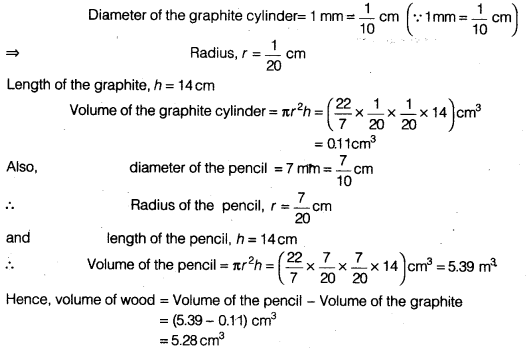Question 8.
A patient in a hospital is given soup daily in a cylindrical bowl of diameter 7 cm. If the bowl is filled with soup to a height of 4 cm, how much soup the hospital has to prepare daily to serve 250 patients?
Solution:
We have, diameter = 7cm
Radius, r = $$\frac { 7 }{ 22 }$$ cm
h= 4 cm
Capacity of the cylindrical bowl = Volume of the cylinder = πr2h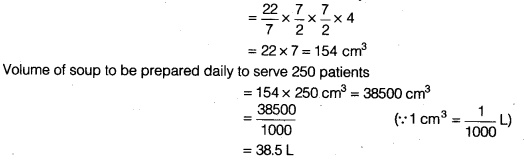We hope the NCERT Solutions for Class 9 Maths Chapter 13 Surface Areas and Volumes Ex 13.6 help you. If you have any query regarding NCERT Solutions for Class 9 Maths Chapter 13 Surface Areas and Volumes Ex 13.6, drop a comment below and we will get back to you at the earliest.